# Supergraph

(diff) ← Older revision | Latest revision (diff) | Newer revision → (diff)

of a function

Letbe a function defined on some setwith values in the extended real line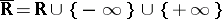. Then the supergraph ofis the subset of the Cartesian productconsisting of all points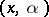lying "above" the graph of. The supergraph is sometimes called the epigraph and is denoted by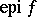:The projection of the supergraph ontois known as the effective domain of, denoted by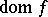: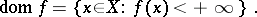The functionis said to be proper if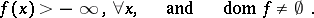A function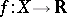defined on a real vector spaceis convex if and only ifis a convex subset of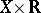. A function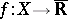defined on a topological spaceis upper semi-continuous if and only ifis a closed set.